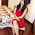## Friday, June 7, 2013

### Indexer Enumerator etc. etc. examples

It is so simple to work with an Array or List of string. But when it comes to use List of complex class objects it becomes nightmare. Some simply prefer to go on implementing long inefficient codes for it. At the same time due to lots of code and complexity quality and scalability impacted.

Lets now give a second thought to it and understand how can we implement it efficiently with minimal effort.

What is an Indexer?

Defining an indexer allows you to create classes that act like "virtual arrays." Instances of that class can be accessed using the [] array access operator. Defining an indexer in C# is similar to defining operator [] in C++, but is considerably more flexible. For classes that encapsulate array- or collection-like functionality, using an indexer allows the users of that class to use the array syntax to access the class. An indexer is often used to implement a stack so that its contents may be accessed without item removal.

An indexer's simple syntax helps client applications access element groups as an array object member (type, class, or struct). An indexer provides an indirect method of inserting boundary checking logic. Due to its intuitive nature, an indexer improves code readability.

Now, in the implementation below there are multiple implementations how we can use Indexer and Collection for simple implementation of complex class / struct objects. A Class is a reference type so the objects needed to created explicitly. Where as a struct in value type and there is no need to create object explicitly.

* First implementation
A simple Test1 class with string array variable and using Indexer exposing string array.

* Second implementation
Extending First implementation to make it two dimensional array.

* Third implementation
Implementation of array using struct, being value type implementation is as simple as siring array. Extending it is easy and so just a simple implementation.

* Fourth implementation
It is simple class array implementation but it not that simple. If you see after defining length of array each node object needed to be created. This is an overhead but if implemented in a better way will be more simpler and scalable.

* Fifth implementation
This is extension of previous implementation but this time created new list class using IEnumerable<> interface and implementing GetEnumerator. This way even though it is complicated for simple implementation but for complicated class object it is simple.

```namespace Indexer
{
class Program
{
static void Main(string[] args)
{
// First implementation
// implementing class using indexer
Console.WriteLine("implementing class using indexer");
Test1 EE = new Test1();
EE = "this 1";
EE = "this 2";
//foreach (var X1 in EE) cannot implement because it doesn't have public definition of GetNumerator
Console.WriteLine("{0}", EE.ToString());
Console.WriteLine("{0}", EE.ToString());

// Second implementation
// extending indexable class to build 2 dimentional array
Console.WriteLine("extending indexable class to build 2 dimentional array");
Test1[] XX = new Test1;
XX = new Test1();
XX = new Test1();
XX = "XXX";
XX = "YYY";
XX = "aaa";
XX = "bbb";
foreach (var X1 in XX)
Console.WriteLine(X1 + " ; " + X1);

// Third implementation
// simple implementation of array of object using struct
Console.WriteLine("simple implementation of array of abject using struct");
objStruct[] ddd = new objStruct;
ddd.iCnt = 1;
ddd.sName = "name 1";
ddd.iCnt = 2;
ddd.sName = "name 2";
foreach (var Xd in ddd)
Console.WriteLine(Xd.iCnt.ToString() + " ; " + Xd.sName);

// Fourth implementation
// simple implementation of array of object using class
Console.WriteLine("simple implementation of array of object using class");
objClass[] sss = new objClass;
sss = new objClass();
sss = new objClass();
sss.iCnt = 1;
sss.sName = "name 1";
sss.iCnt = 2;
sss.sName = "name 2";
foreach (var Xd in sss)
Console.WriteLine(Xd.iCnt.ToString() + " ; " + Xd.sName);

// Fifth implementation
// extending class object to make it enumerable using IEnumerable interface
Console.WriteLine("extending it enumerable using IEnumerable interface");
objClassList ssX = new objClassList();
ssX.Add(new objClass() { iCnt = 1, sName = "XXC" });
ssX.Add(new objClass() { iCnt = 2, sName = "CCC" });
ssX.Add(new objClass() { iCnt = 3, sName = "BBB" });
foreach (var w in ssX)
Console.WriteLine(w.iCnt + " # " + w.sName);
}
}
class Test1
{
private string[] names;
public Test1()
{
this.names = new string;
}
public string this[int i]
{
get { return this.names[i]; }
set { this.names[i] = value; }
}
}
struct objStruct
{
public int iCnt;
public string sName;
}
class objClass
{
public int iCnt;
public string sName;

}
class objClassList : IEnumerable<objclass>
{
private List<objclass> objList = new List<objclass>();
public int Count
{
get
{
return objList.Count;
}
}
public objClass this[int index]
{
get
{
return objList[index];
}
}
{
}
public void Remove(objClass objX)
{
objList.Remove(objX);
}
public IEnumerator<objclass> GetEnumerator()
{
return this.objList.GetEnumerator();
}
System.Collections.IEnumerator System.Collections.IEnumerable.GetEnumerator()
{
return this.GetEnumerator();
}
}
}
```

Console Output
implementing class using indexer
this 1
this 2
extending indexable class to build 2 dimentional array
XXX ; YYY
aaa ; bbb
simple implementation of array of abject using struct
1 ; name 1
2 ; name 2
simple implementation of array of object using class
1 ; name 1
2 ; name 2
extending it enumerable using IEnumerable interface
1 # XXC
2 # CCC
3 # BBB

1.2.3.